### Practice Set 1.3 Similarity Class 10th Mathematics Part 2 MHB Solution

Practice Set 1.3

1. In figure 1.55, ∠ABC = 75°, ∠EDC = 75° state which two triangles are similar and by…
2. Are the triangles in figure 1.56 similar? If yes, by which test?
3. As shown in figure 1.57, two poles of height 8 m and 4 m are perpendicular to the…
4. In ΔABC, AP ⊥ BC, BQ ⊥ AC B- P-C, A-Q - C then prove that, ΔCPA ~ ΔCQB. If AP = 7, BQ =…
5. Given : In trapezium PQRS, side PQ || side SR, AR = 5AP, AS = 5AQ then prove that, SR =…
6. In trapezium ABCD, (Figure 1.60) side AB || side DC, diagonals AC and BD intersect in…
7. □ ABCD is a parallelogram point E is on side BC. Line DE intersects ray AB in point T.…
8. In the figure, seg AC and seg BD intersect each other in point P and ap/cp = bp/dp…
9. In the figure, in ΔABC, point D on side BC is such that, ∠BAC = ∠ADC. Prove that, CA^2…

###### Practice Set 1.3
Question 1.

In figure 1.55, ∠ABC = 75°, ∠EDC = 75° state which two triangles are similar and by which test? Also write the similarity of these two triangles by a proper one to one correspondence.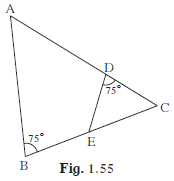With one- to-one correspondence ABC ↔ EDC

∵ ∠ABC ≅ ∠EDC = 75°

∠ACB ≅ ∠ECD (Is common in both the triangles ABC and EDC)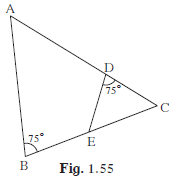⇒ Δ ABC~Δ EDC ………(By AA Test)

Question 2.

Are the triangles in figure 1.56 similar? If yes, by which test?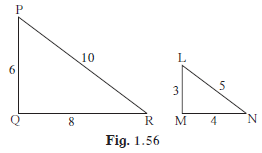In Δ PQR and Δ LMN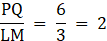And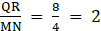And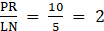⇒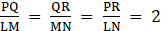⇒ ΔPQR~ΔLMN …………(By SSS Similarity Test)

Question 3.

As shown in figure 1.57, two poles of height 8 m and 4 m are perpendicular to the ground. If the length of shadow of smaller pole due to sunlight is 6 m then how long will be the shadow of the bigger pole at the same time?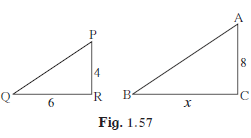∵ the shadows are measured at the same time

⇒ angle of of elevation will be equal for both the pole

⇒ Δ PQR~Δ ABC ………(By AA Test)

⇒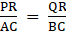⇒ BC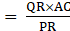⇒ x =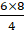⇒ x = 12 m

Question 4.

In ΔABC, AP ⊥ BC, BQ ⊥ AC B- P-C, A-Q - C then prove that, ΔCPA ~ ΔCQB. If AP = 7, BQ = 8, BC = 12 then find AC.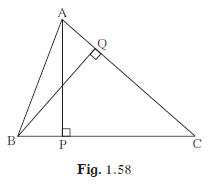From fig.

⇒ ∠ APC≅ ∠ BQC (∵ AP⊥ BC and BQ⊥ AC)

⇒ Also, ∠ ACP≅ ∠ BCQ (Common)

⇒ Δ CPA~Δ CQB (By AA Test)

⇒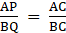⇒ AC =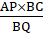⇒AC =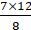⇒AC = 10.5

Question 5.

Given : In trapezium PQRS, side PQ || side SR, AR = 5AP, AS = 5AQ then prove that, SR = 5PQ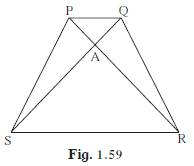Given that, AR = 5AP and AS = 5AQ

⇒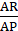= 5 ………(1)

And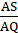= 5……….(2)

⇒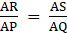And, ∠ SAR≅ ∠ QAP …… (opposite angles)

⇒ Δ SAR ~ Δ QAP …………(SAS Test of similarity)

⇒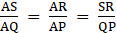(corresponding sides are proportional)

But,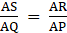= 5

⇒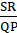= 5

⇒ SR = 5PQ

Question 6.

In trapezium ABCD, (Figure 1.60) side AB || side DC, diagonals AC and BD intersect in point O. If AB = 20, DC = 6, OB = 15 then find OD.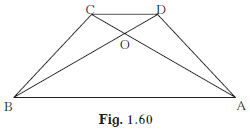In Δ AOB and ΔCOD

⇒ ∠ AOB≅ ∠ COD (opposite angles)

⇒ ∠ CDO≅ ∠ ABO (Alternate angles ∵ AB||DC)

⇒ Δ AOB ~ Δ COD (By AA Test)

⇒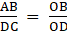(corresponding sides are proportional)

⇒ OD =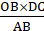⇒ OD =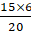⇒ OD = 4.5

Question 7.

□ ABCD is a parallelogram point E is on side BC. Line DE intersects ray AB in point T. Prove that DE × BE = CE × TE.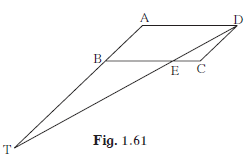In Δ CED and ΔBET

⇒ ∠ CED≅ ∠ BET (opposite angles)

⇒ ∠ CDE≅ ∠ BTE (Alternate angles)

(∵ AB||DC ⇒ BT||DC, as BT is extension to AB)

⇒ Δ CED ~ Δ BET (By AA Test)

⇒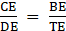(corresponding sides are proportional)

⇒ DE× BE = CE×TE

Question 8.

In the figure, seg AC and seg BD intersect each other in point P and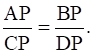Prove that, ΔABP ~ ΔCDP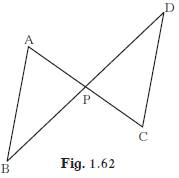In Δ APB & Δ CPD

⇒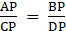……(Given)

And, ∠APB = ∠DPC (vertically opposite angles)

⇒ Δ APB ~ Δ CPD (By SAS Test)

Question 9.

In the figure, in ΔABC, point D on side BC is such that, ∠BAC = ∠ADC.

Prove that, CA2 = CB × CD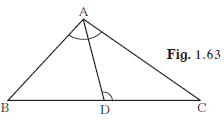In Δ BAC & Δ ADC

⇒ ∠ BAC ≅ ∠ ADC ……(Given)

And, ∠ACB ≅ ∠DCA ……(common)

⇒ Δ BAC ~ Δ ADC (By AA Test)

⇒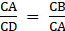(corresponding sides are proportional)

⇒ CA2 = CB×CD

## PDF FILE TO YOUR EMAIL IMMEDIATELY PURCHASE NOTES & PAPER SOLUTION. @ Rs. 50/- each (GST extra)

SUBJECTS

HINDI ENTIRE PAPER SOLUTION

MARATHI PAPER SOLUTION
SSC MATHS I PAPER SOLUTION
SSC MATHS II PAPER SOLUTION
SSC SCIENCE I PAPER SOLUTION
SSC SCIENCE II PAPER SOLUTION
SSC ENGLISH PAPER SOLUTION
SSC & HSC ENGLISH WRITING SKILL
HSC ACCOUNTS NOTES
HSC OCM NOTES
HSC ECONOMICS NOTES
HSC SECRETARIAL PRACTICE NOTES

2019 Board Paper Solution

HSC ENGLISH SET A 2019 21st February, 2019

HSC ENGLISH SET B 2019 21st February, 2019

HSC ENGLISH SET C 2019 21st February, 2019

HSC ENGLISH SET D 2019 21st February, 2019

SECRETARIAL PRACTICE (S.P) 2019 25th February, 2019

HSC XII PHYSICS 2019 25th February, 2019

CHEMISTRY XII HSC SOLUTION 27th, February, 2019

OCM PAPER SOLUTION 2019 27th, February, 2019

HSC MATHS PAPER SOLUTION COMMERCE, 2nd March, 2019

HSC MATHS PAPER SOLUTION SCIENCE 2nd, March, 2019

SSC ENGLISH STD 10 5TH MARCH, 2019.

HSC XII ACCOUNTS 2019 6th March, 2019

HSC XII BIOLOGY 2019 6TH March, 2019

HSC XII ECONOMICS 9Th March 2019

SSC Maths I March 2019 Solution 10th Standard11th, March, 2019

SSC MATHS II MARCH 2019 SOLUTION 10TH STD.13th March, 2019

SSC SCIENCE I MARCH 2019 SOLUTION 10TH STD. 15th March, 2019.

SSC SCIENCE II MARCH 2019 SOLUTION 10TH STD. 18th March, 2019.

SSC SOCIAL SCIENCE I MARCH 2019 SOLUTION20th March, 2019

SSC SOCIAL SCIENCE II MARCH 2019 SOLUTION, 22nd March, 2019

XII CBSE - BOARD - MARCH - 2019 ENGLISH - QP + SOLUTIONS, 2nd March, 2019

HSC Maharashtra Board Papers 2020

(Std 12th English Medium)

HSC ECONOMICS MARCH 2020

HSC OCM MARCH 2020

HSC ACCOUNTS MARCH 2020

HSC S.P. MARCH 2020

HSC ENGLISH MARCH 2020

HSC HINDI MARCH 2020

HSC MARATHI MARCH 2020

HSC MATHS MARCH 2020

SSC Maharashtra Board Papers 2020

(Std 10th English Medium)

English MARCH 2020

HindI MARCH 2020

Hindi (Composite) MARCH 2020

Marathi MARCH 2020

Mathematics (Paper 1) MARCH 2020

Mathematics (Paper 2) MARCH 2020

Sanskrit MARCH 2020

Important-formula

THANKS